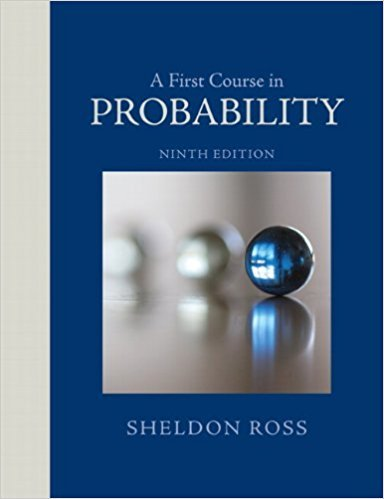×
Get Full Access to A First Course In Probability - 9 Edition - Chapter 4 - Problem 13ste
Get Full Access to A First Course In Probability - 9 Edition - Chapter 4 - Problem 13ste

×

# Each of the members of a 7-judge panel independently makesISBN: 9780321794772 63

## Solution for problem 13STE Chapter 4

A First Course in Probability | 9th Edition

• Textbook Solutions
• 2901 Step-by-step solutions solved by professors and subject experts
• Get 24/7 help from StudySoup virtual teaching assistantsA First Course in Probability | 9th Edition

4 5 1 240 Reviews
25
2
Problem 13STE

Problem 13STE

Each of the members of a 7-judge panel independently makes a correct decision with probability .7. If the panel’s decision is made by majority rule, what is the probability that the panel makes the correct decision? Given that 4 of the judges agreed, what is the probability that the panel made the correct decision?

Step-by-Step Solution:
Step 1 of 3

Solution 13STE

Step1 of 3:

Let us consider a random variable ‘X’ it presents the number of judges with correct decision.

Also, we have n = 7, and p = 0.7.

We need to find the probability that the panel makes the correct decision by using majority rule. And by using Baye’s Theorem.

Step2 of 3:

Here X follows a binomial distribution with parameters ‘n and p.’ the probability mass function of the binomial distribution is:x = 0,1,2,...,n.

The majority of judges is agreed that correct decision, Then assume that judges 1,2, and 3 are not agreed and judges 4,5,6,and 7 agreed.where,is obtained from Excel by using the function “=binomdist(X,n,p,false)”

 X P(X=4) 4 0.226895 5 0.317652 6 0.247063 7 0.082354 Total 0.873964

Therefore, P(Correct decision) = 0.8739 [by using majority rule].

Step3 of 3:

Consider Baye’s Theorem,Where,Therefore,Therefore, P(4 Agreed) = 0.3242.

Now,Therefore, The probability that the panel makes the correct decision is 0.70.

Step 2 of 3

Step 3 of 3

##### ISBN: 9780321794772

Unlock Textbook Solution